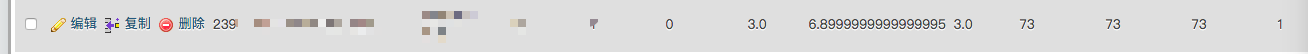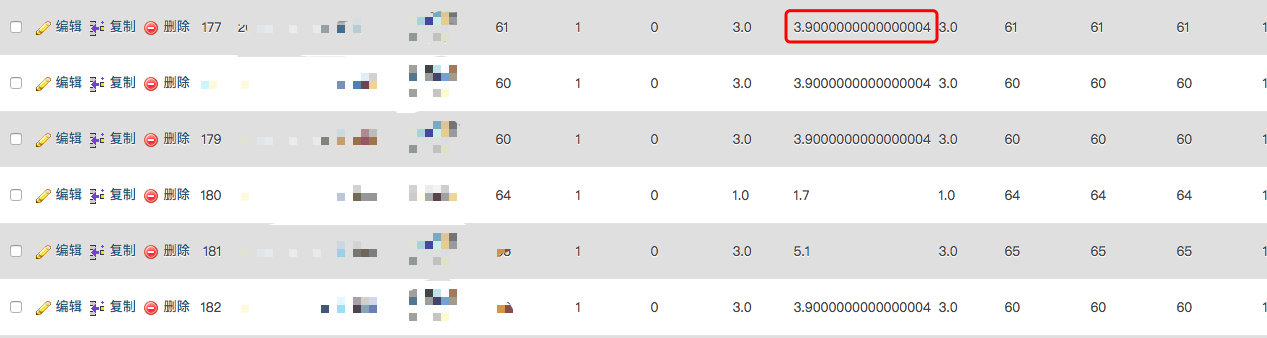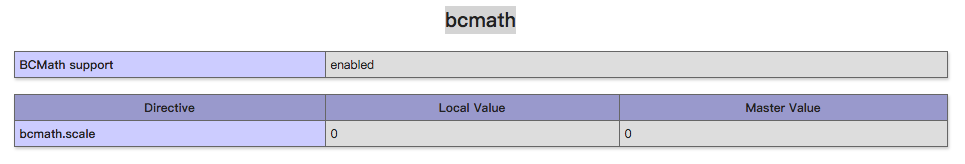﻿ PHP加减乘除出现错误 - 艾超博客 | 勿忘初心，方得始终

## 前言## PHP语言的解决方法

PHP里边编译有叫bcmath的模块，这个是专门用来解决这个问题的。• 加法：bcadd

string bcadd ( string $left_operand , string$right_operand [, int $scale ] )  • 减法：bcsub string bcsub ( string$left_operand , string $right_operand [, int$scale = int ] )

• 乘法：bcmul

string bcmul ( string $left_operand , string$right_operand [, int $scale = int ] )  • 除法：bcdiv string bcdiv ( string$left_operand , string $right_operand [, int$scale = int ] )


## 其它语言的解决方法